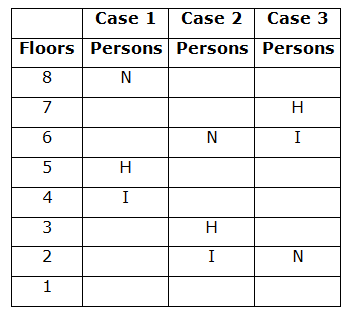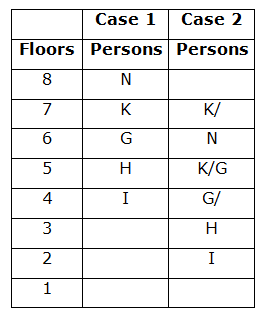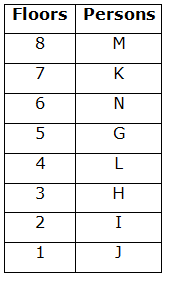# SBI Clerk Prelims Reasoning Ability Questions 2021 (Day-85)

Dear Aspirants, Our IBPS Guide team is providing new series of Reasoning Questions for SBI Clerk Prelims 2021 so the aspirants can practice it on a daily basis. These questions are framed by our skilled experts after understanding your needs thoroughly. Aspirants can practice these new series questions daily to familiarize with the exact exam pattern and make your preparation effective.

Start Quiz

Number series

Direction (1-5): Following questions are based on the five three-digit numbers given below:

356   785   987   495   673

1) If all the numbers are arranged in ascending order from left to right then which of the following will be the sum of the first number and second number from the left end?

A.860

B.859

C.851

D.844

E.None of these

2) If the positions of the second and third digits of each of the numbers are interchanged, then what will be the difference of the number which is second from the left end and second from the right end?

A.287

B.299

C.291

D.297

E.None of these

3) If all the numbers are arranged in descending order from left to right then which of the following will be the sum of the square of the first and last digits of the number which is second from the right end?

A.58

B.56

C.43

D.42

E.41

4) If the positions of the first and third digits of each of the number interchange, what will be the difference of the highest and lowest number thus formed?

A.531

B.426

C.413

D.648

E.None of these

5) If the positions of the first and second digits of each of the number interchange, what will be the sum of the second digit of second number from the right end and third digit of the third number from the left end?

A.9

B.8

C.11

D.12

E.None of these

Floor puzzle

Direction (6-10): Study the following information carefully and answer the questions given below:

Eight persons i.e., G, H, I, J, K, L, M and N live on separate floors of 8-floor building such as ground floor is numbered as 1st floor, just above floor it is numbered as 2nd floor and so on till the topmost floor is numbered as 8th floor but not necessarily in the same order.

Three persons live between N and I who lives on an even numbered floor. H lives just above I’s floor. The number of person lives between H and G is same as persons live between G and K. G lives just above L. I lives below G’s floor. M lives on an even numbered floor.

6) How many floors gap is there between K and I?

A.One

B.Two

C.Three

D.More than three

E.None

7) Four of the following five are alike in a certain way based on the final arrangement, find the one that does not belong to that group?

A.J

B.H

C.G

D.M

E.K

8) Which of the following pair of persons live on an odd numbered floor?

A.J,N

B.K,M

C.G,H

D.I,L

E.None of these

9) Who among the following lives on 6th floor?

A.M

B.N

C.I

D.L

E.None of these

10) Which of the following statement is false?

A.M lives on topmost floor

B.K lives on an odd numbered floor

C.More than three persons live between N and J

D.More than two persons live between G and I

E.None is false

After arranging in ascending order from left to right, we get

356  495  673  785  987

Hence the sum of the first number and second number from left end = 356+495 = 851.

After interchanging the digit, we get

365 758 978 459 637

Hence the difference of the number which is second from the left end and second from the right end = 758 – 459 = 299.

After arranging it in descending order from left to right, we get

987 785 673 495 356

Hence the sum of the square of the first and last digits of the number which is second from right end= 42 + 52= 41.

After interchanging the digits, we get

653 587 789 594 376

Hence the difference between the highest and lowest number thus formed = 789 – 376 = 413.

After interchanging the digits, we get

536 875 897 945 763

Hence the sum of the second digit of the second number from the right end and third digit of the third number from the left end = 4 + 7 = 11.

Directions (6-10) :

As per the given statements, three persons live between N and I who lives on an even numbered floor. H lives just above I’s floor. So here we have three possible cases i.e., Case 1, Case 2 and Case 3.The number of person lives between H and G is same as persons live between G and K. I lives below G’s floor. So Case 3 gets eliminated here.G lives just above L. So, Case 1 gets eliminated. M lives on an even numbered floor